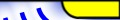Peak to average power ratio reduction of OFDM signals using Peak Reduction Carriers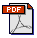Print Friendly Version in PDF (76KB)
 E. Lawrey, C. J. Kikkert Electrical and Computer Engineering James Cook University, Douglas Campus, Townsville, Queensland, 4814, Australia Email: ofdm@skydsp.com, Keith.Kikkert@jcu.edu.au Published: Fifth International Symposium on Signal Processing and its Applications, ISSPA ’99, Brisbane, Australia, 22-25 August, 1999 Organised by the Signal Processing Research Centre, QUT, Brisbane, Australia

## Abstract

A technique is described for a better than 5 dB reduction in the Peak to Average Power Ratio (PAPR) of an OFDM signal. The optimal amplitude and phase of additional peak reduction carriers (PRC) is obtained using a code-book, which is obtained using a search of all possible signal combinations.

## 1 Introduction

Wireless digital communications is rapidly expanding, resulting in a demand for wireless systems that are reliable and have a high spectral efficiency. Orthogonal Frequency Division Multiplexing (OFDM) has a high tolerance to multipath signals and is spectrally efficient making it a good candidate for future wireless communication systems.

One disadvantage of OFDM is that the peak of the signal can be up to N times the average power (where N is the number of carriers). These large peaks increase the amount of intermodulation distortion resulting in an increase in the error rate. The average signal power must be kept low in order to prevent the transmitter amplifier limiting. Minimising the PAPR allows a higher average power to be transmitted for a fixed peak power, improving the overall signal to noise ratio at the receiver. It is therefore important to minimise the PAPR.

A large amount of research has been done on broadcast OFDM systems, however most wireless communication systems must support multiple users. One application that a multi-user OFDM system would be suitable for is fixed wireless telephony applications. In such a system each user is allocated a small percentage of the system carriers, typically 4-16 depending on the symbol rate used. This paper presents a method for reducing the PAPR of a OFDM signal that contains data. It is optimised for a low number of carriers making it a suitable technique for multi-user OFDM.

## 2 Background

The PAPR of an OFDM signal can be reduced in several ways. Selective mapping [1, 2] involves generating a large set of data vectors all representing the same information. The data vector with the lowest resulting PAPR is selected. Information about which particular data vector was used is sent as additional carriers. However there may be potential problems with decoding the signal in the presence of noise with selective mapping. Errors in the reverse mapping would result in the data of whole symbols being lost.

Another technique similar to selective mapping is using Golay sequences . Information is transmitted by mapping each data word with a Golay sequence. Using Golay sequences result in a low PAPR, typically 3-6 dB, however the coding rate is poor, typically half, resulting in a large bandwidth increase. Cyclic coding  involves adding extra carriers in which the phase of every fourth carrier is calculated based on an algorithm using the phase of the previous three information carriers. This method is similar to the presented technique except that it gives sub optimal results.

## 3 Peak Reduction Carriers

This paper presents a technique that combines selective mapping and cyclic coding. A reduction in the PAPR is achieved by adding extra carriers referred to as Peak Reduction Carriers (PRC). The phase and amplitude of the PRCs is varied to minimise the overall PAPR. The original information carriers are unaffected and can be decoded normally. The receiver can disregard the PRCs, or they can be used for error detection. The frequency of PRCs, or relative positioning of the PRCs can be varied with respect to the information carriers depending on the application. The results presented were found using a computationally intensive exhaustive search to find the optimal setting for the PRCs. However it is assumed that further work will allow a more efficient algorithm to be found.

An optimal setting for the PRCs corresponds to the combination of phase and amplitude that achieves the lowest PAPR of the overall OFDM symbol (information carriers and PRCs). In this paper the phase and amplitude of the PRCs was set in a coarse quantised manner to minimise the number of combinations needed to be searched. The phase of the PRCs was set to 0° or 180° and the carriers were turned on or off. There are therefore 3M combinations for the PRCs for each information code word (where M is the number of PRCs). This level of quantisation was found to be appropriate for BPSK information carriers. Finer quantisation may produce improved results for higher modulation schemes.

An exhaustive search of all combinations of allowable phase and amplitude gives optimal PRCs, but is computationally intensive. This method can be used for small numbers of carriers where the optimal PRC coding can be stored in a look up table or code-book. This is impractical for more than 16 information carriers or for more than 10 PRCs as the number of combinations becomes too large to store and calculate. However for some multi-user OFDM applications 16 or less carriers per user is sufficient.

The results shown were calculated based on all combinations of information code words, thus will give a good indication of the practical PAPR improvement.

For each experiment the inverse fast Fourier transform (IFFT) of the carrier configuration was used to give a complex base band signal. Let the complex base band signal be defined as in eqn. 1.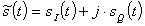(1)
When this is quadrature modulated to RF the signal can be written in polar form as: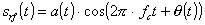(2)
where a(t) is the amplitude and q(t) is the phase of the signal. Thus: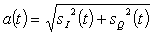(3)
The definition of the PAPR in eqn 4, where T is the OFDM symbol period, can be used for RF as well as base band .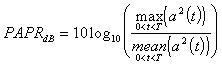(4)
For the simulations carried out, the base band carriers were centred on DC and the size of the IFFT was made at least 8 times greater than the number of carriers, oversampling the time domain signal. This ensures that peaks in the signal were accurately represented to get an accurate PAPR [4, 5].

## 3.1 Results

The simplest arrangement for the relative positioning of the data and PRCs is to have a block of data carriers immediately followed by a block of PRCs. This arrangement was used for the results shown in figures 1-3.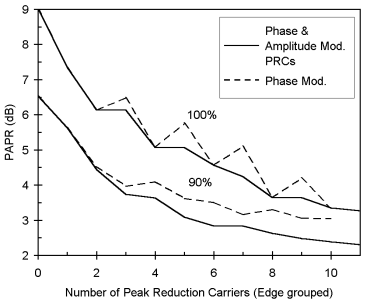Figure 1.  PAPR verses number of edge grouped PRCs (8 BPSK data carriers)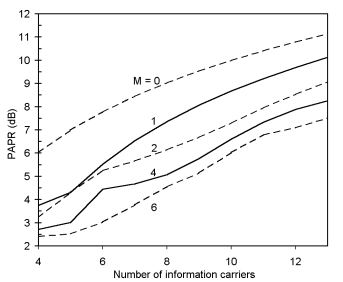Figure 2.  Maximum PAPR verses number of data carriers and edge grouped PRCs, where M is the number of PRCs.

Figure 1 shows the worst case PAPR and the 90% point in the cumulative distribution of PAPR as the number of PRCs is increased. The maximum PAPR for the 8 information carriers is reduced by >5.5dB for the addition of 10 PRCs. Selecting the optimal amplitude and phase of the PRC improves the performance significantly as compared with only setting the phase as used in cyclic coding . For this reason phase and amplitude modulation of the PRCs was used in all later experiments due to the improved performance.

Figure 2 shows the effect of adding PRCs to the PAPR as the number of information carriers is varied. The improvement in PAPR remains relatively constant as the number of information carriers is increased. This shows that this technique gives consistent performance gains as the number of information carriers is varied.

Adding PRCs reduces the PAPR at the expense of additional transmission power. Figure 3 shows the net improvement in PAPR due to the addition of PRCs. The PAPR reduction was calculated as the difference between the PAPR results for zero PRCs and the PAPR results with the addition of PRCs. The loss in signal power due to the PRCs was then subtracted from the PAPR reduction in order to give the net PAPR improvement. If the data signal power lost due to the transmission of the PRCs was more than the PAPR gain then there would be little point in adding the PRCs. It can be seen that for 10 BPSK carriers there is little improvement in adding more than 2 PRCs. In fact adding more than 5 PRCs results in a worsening of the average (50%) PAPR. This is due to the power cost of the PRCs.

## 4 Effect of PRC Position

Previous results are shown for grouped PRCs that were positioned immediately after the data carriers as shown in Figure 4a. Two different positioning tests were performed. One test kept the PRCs grouped together as in Figure 4a, however they were moved with respect to the data carriers as in Figure 4b.The second test positioned the PRCs in a spread out manner. The best spread pattern was established using a randomised search.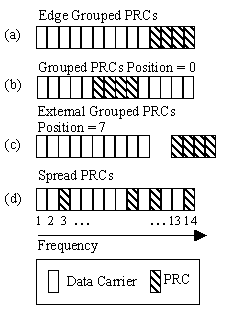Figure 4.  PRC position combinations
Adding PRCs use a significant amount of additional bandwidth. It is therefore important to minimise the number used, or to position the PRCs so that the bandwidth can be reused. For example, in a multi-user OFDM system where each user transmits a block of carriers, the PRCs can be overlapped, i.e. they are transmitted at the same frequency, effectively halving the bandwidth.

## 4.1 Grouped PRCs

In this scheme the PRCs were maintained as a group of carriers. They were repositioned by sliding them with respect to the data carriers. Figure 5 and 6 show the effect of the position on effectiveness of the PRCs. Figure 5 shows for a small number of PRCs the position of the PRCs within the data carriers has little effect on the performance. However with 4 or more PRCs the position has a significant effect on the performance of the PRCs.Placing the PRCs within the data carriers with an off centre of 3 carriers gives the best results. This gives a further reduction of 1dB as compared with edge grouped PRCs. Not having edge grouped PRCs would prevent overlapping of the PRCs for a multi-user OFDM, thus doubling the bandwidth used by the PRCs.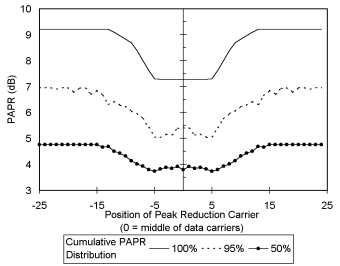Figure 5.  PAPR verses position of 2 grouped PRCs
(10 BPSK data carriers)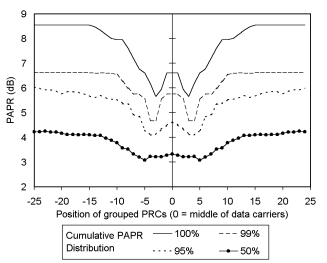Figure 6.  PAPR verses position of 4 grouped PRCs (10 BPSK data carriers)

The position of the 4 grouped PRCs had a significant effect on the PRC performance, thus it seemed likely that spreading the PRCs out might lead to further improvements. The exact relationship between the position of the PRCs and the PAPR distribution is currently unknown and so a random search was used for optimisation. The PRCs and data carriers were positioned randomly to form a block of carriers with no gaps as shown in figure 4d. For each position combination the PAPR distribution was found and the combination which resulted in the lowest maximum PAPR was selected as the optimised PRCs position.

The PAPR distribution was found by testing all combinations of the data code words. For each data code word combination the optimum PRCs were found as described in section 3. The PAPR distribution verses the number of PRCs is shown in figure 7. This result is for 10 data carriers and shows that spreading the PRCs can result in large reductions in the PAPR of the OFDM symbol. A reduction of > 6dB is possible.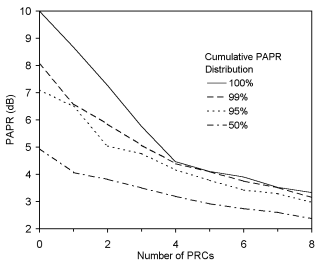Figure 7.  PAPR verses the number of spread PRCs (10 BPSK data carriers)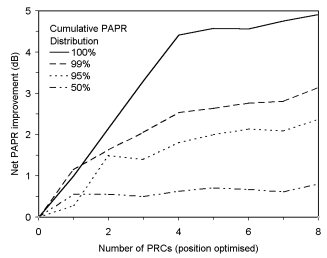Figure 8.  Net improvement in PAPR, position optimised PRCs (10 BPSK data carriers)

Figure 8 shows the overall net improvement in the PAPR using position optimised PRC. This can be directly compared to figure 3 which shows the results for edge grouped PRCs. The maximum net gain for position optimised PRCs is nearly double that of the edge grouped PRCs. Figure 8 shows that the net PAPR gain increases rapidly up to 4 PRCs, after which the gain is minimal. Thus the optimal number of PRCs would be 4 for 10 data carriers. Other tests also show that the number of PRCs needs to be approximately 40% of the number of data carriers in order to get significant improvements in the PAPR.

Overlapping of the spread PRCs in a multi-user OFDM system is more difficult as most of the PRCs will be bounded with data carriers. Thus simple overlapping may not be possible. This would be the case if the data and PRC are grouped. However if the carriers for each user are spread out it might be possible have spread out PRCs that overlap between the users, but still provide a large reduction in the PAPR.

The addition of 4 PRCs with 10 data carriers results is a large net gain of 4.5 dB, allowing more power to be transmitted. For a transmission with no PRCs at an error rate of 1x10-3, adding the PRCs and maintaining the same peak power the error rate would be decreased to 1x10-7 . This is more efficient than adding error correcting bits at the same coding rate. For example using Hamming coding at a rate of 4 parity bits for 11 data bits gives a gain of only 1.2 dB at a bit error rate of 1x10-4  which is significantly less than 4.5dB.

Table 1 shows the number PRC positions tested, and the best combination found.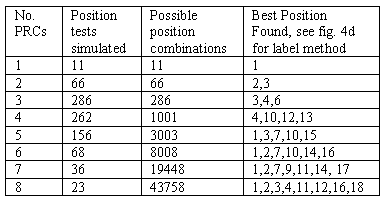Table 1.  Optimised positions found for 10 BPSK data carriers

## 5 Conclusion

Adding peak reduction carriers can significantly improve the PAPR of an OFDM signal. The PRCs can result in a reduction of >6dB in the maximum PAPR and a net reduction of >4.5dB when the additional power for PRCs is taken into account. It was found that varying the amplitude as well as phase for the PRCs gave improved performance over just phase variation. It was also found that spreading the position of the PRCs resulted in a better performance than grouped PRCs. Adding more PRCs results in a lower PAPR, however the use of large numbers of PRCs is limited by the cost of additional transmission power, bandwidth and complexity limits.

## 6 References

1. Bauml, R.W., Fischer, R.F.H., Huber, J.B., Reducing the peak-to-average power ratio of multicarrier modulation by selected mapping, Electronic Letters, 1996, Vol. 32, pp. 2056-2057

2. Van Eetvelt, J., Wade, G., Tomlinson, M., Peak to average power reduction for OFDM schemes by selective scrambling, Electronic Letters, 1996, Vol. 32, pp. 1963-1964

3. Wulich, D., Reduction of peak to mean ratio of multicarrier modulation using cyclic coding, Electronic Letters, 1996, Vol. 32, pp. 432-433

4. Tellambura, C., Use of m-sequences for OFDM peak-to-average power ratio reduction, Electronic. Letters, 1997, Vol. 33, pp. 1300-1301

5. Tellambura, C., Phase optimisation criterion for reducing peak-to-average power ratio in OFDM, Electronic Letters, 1998, Vol. 34, pp. 169-170

6. Davis, J.A., Jedwab, J., Peak-to-mean power control and error correction for OFDM transmission using Golay sequences and Reed-Muller codes, Electronic Letters, 1997, Vol. 33, pp. 267-268

7. Sklar, B., Digital Communications Fundamentals and Applications, Prentice Hall, 1988, pp. 300